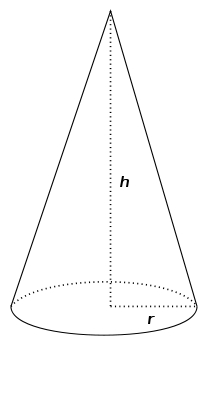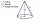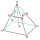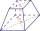# From plasticine

Michael modeled from plasticine a 15 cm high pyramid with a rectangular base with the sides of the base a = 12 cm and b = 8 cm. From this pyramid, Janka modeled a rotating cone with a base diameter d = 10 cm. How tall was Janka's cone?

h2 =  18.3346 cm

### Step-by-step explanation:Did you find an error or inaccuracy? Feel free to write us. Thank you!Tips to related online calculators
Tip: Our volume units converter will help you with the conversion of volume units.

#### You need to know the following knowledge to solve this word math problem:

We encourage you to watch this tutorial video on this math problem:

## Related math problems and questions:Calculate the surface of a quadrilateral pyramid, which has a rectangular base with dimensions a = 8 cm, b = 6 cm and height H = 10 cm.A quadrilateral pyramid, which has a rectangular base with dimensions of 24 cm, 13 cm. The height of the pyramid is 18cm. Calculate 1/the area of the base 2/casing area 3/pyramid surface 4/volume of the pyramid
• A plasticineJožko modeled from plasticine. He used 27g of plasticine to model a 3 cm long cube. How many grams of plasticine will it need to mold cubes with an edge of 6cm?The quadrilateral pyramid has a rectangular base of 24 cm x 3.2dm and a body height of 0.4m. Calculate its volume and surface area.
• Rotating coneFind the rotating cone's surface and volume if its side is 150 mm long and the circumference of the base is 43.96 cm.
• The volumeThe volume of the rotating cone is 376.8cm³, the height of this cone is 1dm. Calculate the diameter of the cone base.
• ConeThe rotating cone volume is 9.42 cm3, with a height 10 cm. What angle is between the side of the cone and its base?We have a regular quadrilateral pyramid with a base edge a = 10 cm and a height v = 7 cm. Calculate 1/base content 2/casing content 3/pyramid surface 4/volume of the pyramid
• The base 2The base diameter of a right cone is 16cm and it's slant height is 12cm. A. ) Find the perpendicular height of the cone to 1 decimal place. B. ) Find the volume of the cone, convert to 3 significant figure. Take pi =3.14What is the volume of a regular quadrilateral pyramid if its surface is 576 cm2 and the base edge is 16 cm?In a regular quadrilateral pyramid, the height is 6.5 cm and the angle between the base and the side wall is 42°. Calculate the surface area and volume of the body. Round calculations to 1 decimal place.It is given a regular tetrahedral pyramid with a base edge of 6 cm and the height of the pyramid 10 cm. Calculate the length of its side edges.Circular cone of height 15 cm and volume 5699 cm3 is at one-third of the height (measured from the bottom) cut by a plane parallel to the base. Calculate the radius and circumference of the circular cut.A cone with a base radius of 10 cm and a height of 12 cm is given. At what height above the base should we divide it by a section parallel to the base so that the volumes of the two resulting bodies are the same? Express the result in cm.The truncated regular quadrilateral pyramid has a volume of 74 cm3, a height v = 6 cm, and an area of the lower base 15 cm2 greater than the upper base's content. Calculate the area of the upper base.The height of a regular quadrilateral prism is v = 10 cm, the deviation of the body diagonal from the base is 60°. Determine the length of the base edges, the surface, and the volume of the prism.Michaela has two vases in her collection. The first vase has the shape of a cone with a base diameter d = 20 cm; the second vase has the shape of a truncated cone with the lower base d1 = 25 cm and with the diameter of the upper base d2 = 15 cm. Which vas